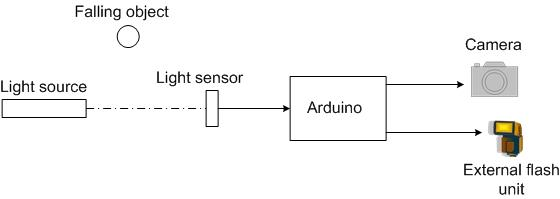Capture the image of a falling object

The aim of this project is to create a setup to capture the image of a falling object or any object in motion at a precise time using a DSLR and Arduino microcontroller. It can be done in many different ways, but the method I tried is illustrated below.The idea is, when the object falling or moving blocks the light source from reaching light sensor the camera and/or the external flash unit is triggered. An Arduino Uno microcontroller board is used to measure the sensor values and send trigger signal to the camera/flash. With the microcontroller you can change the threshold value of the light sensor value and add precise dealy to the trigger signal. Here the light source is a simple laser pointer is used as a light source and a cadmium sulphide photocell acts as a light sensor. The wiring diagram is shown below,A bit of explanation on how this circuit works. The photocells or the Light Dependent Resistors (LDR) have a high resistance when there is no light, equivalent to open circuit. When light is shine on them their resistance reduces. The value of the resistance depends on how bright the light is. Initially when the light source is pointed to the LDR, it has a low resistance. The LDR and the resitor R1 act as a voltage divider circuit. The voltage drop across R1 is measured by the analog input terminal of the Arduino board. Ideally would have almost no resistance (0 ohms) when a bright light is focused on it. Which means the voltage drop measured across R1 would be ~5V. But depending on the intensity of the light source and the distance from the LDR, the LDR will have some low resistance value and hence the voltage across R1 (measured at A0 terminal) will be less than 5V. When a moving object cuts the beam of light the LDR value increases and hence the voltage across R1 drops. By comparing this value with an appropriate threshold value (which you can set to be somewhere between when the light is on the LDR and when it is blocked by the object), you can use the digital output lines to trigger the camera and/or flash by setting it to high.

READ  Capture the image of a falling object using Arduino

For more detail: Capture the image of a falling object

This Post / Project can also be found using search terms:

• falling object arduino